# Horner scheme

(diff) ← Older revision | Latest revision (diff) | Newer revision → (diff)

A method for obtaining the incomplete fraction and the remainder in the division of a polynomial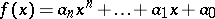(1)

by a binomial, where all the coefficients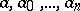lie in a certain field, e.g. in the field of complex numbers. Any polynomialcan be uniquely represented in the form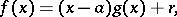where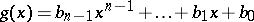is the incomplete fraction, whileis the remainder which, according to the Bezout theorem, equals. The coefficients of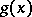andare calculated by the recurrence formulas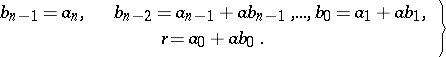(2)

The following table:'

<tbody> </tbody>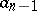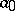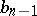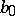whose upper line is given, while the lower line is filled in accordance with the formulas (2), is used in the computations. In fact, this method is identical with the method of Ch'in Chiu-Shao employed in medieval China. At the beginning of the 19th century it was rediscovered, almost simultaneously, by W.G. Horner  and P. Ruffini .

  W.G. Horner, Philos. Transact. Roy. Soc. London Ser. A , 1 (1819) pp. 308–335  P. Ruffini, Mem. Coronata Soc. Ital. Sci. , 9 (1802) pp. 44–526

Horner's scheme is used for the efficient evaluation of polynomials. The straightforward evaluation offor a given valueby computing the powers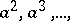and forming the linear combination according to (1) would require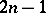multiplications. However, by writingin the "nested" form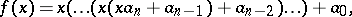only(sequential) multiplications are involved. It is easily verified that using this nested representation the work consists of the computation steps given by (2). Since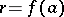, the evaluation of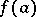requiresinstead ofmultiplications.